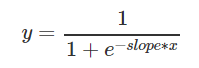Over a million developers have joined DZone.{{announcement.body}}
{{announcement.title}}

# Neural Network Activation Functions From a Programmer's Perspective

DZone 's Guide to

# Neural Network Activation Functions From a Programmer's Perspective

### Dive deeper into how to easily program a neural network in Java by learning about the different types of activation functions.

· AI Zone ·
Free Resource

Comment (3)

Save
{{ articles.views | formatCount}} Views

This post is the second part of series of articles discussing an approach to programming a neural network using Java in a simple and understandable way.

In the previous post, we defined the main components of a neural network and designed them using Java as a programming language. In this post, we will dive deeper into the activation function (also called transfer function) as part of the neural networks.

To recall from the first part of this series, an artificial neuron is a signal collector in the inputs and an activation unit in the output triggering a signal that will be forwarded to other neurons as shown in the following picture:The artificial neuron receives one or more inputs and sums them to produce an output or activation. Usually, the sums of each node are weighted and the sum is passed through an activation function. In most cases, it is the nonlinear activation function that allows such networks to compute nontrivial problems using only a small number of nodes.

In biologically inspired neural networks, the activation function is usually an abstraction representing the rate of action potential firing in the cell. Using Java as a programming language, this abstraction can be designed using an activation function interface:

/**
* Neural network's activation function interface.
*/
public interface ActivationFunction {

/**
* Performs calculation based on the sum of input neurons output.
*
* @param summedInput
*            neuron's sum of outputs respectively inputs for the connected
*            neuron
*
* @return Output's calculation based on the sum of inputs
*/
double calculateOutput(double summedInput);

}

The implementations of this interface will provide a way to easily experiment with and replace various types of activation functions. Let's start implementing them!

## Step Function

The simplest form of activation function is binary — the neuron is either firing or not. The output y of this activation function is binary, depending on whether the input meets a specified threshold, θ. The "signal" is sent, i.e. the output is set to one if the activation meets the threshold.This function is used in perceptrons. It performs a division of the space of inputs by a hyperplane. It is especially useful in the last layer of a network intended to perform binary classification of the inputs.

/**
* Step neuron activation function, the output y of this activation function is
* binary, depending on whether the input meets a specified threshold, 0. The
* "signal" is sent, i.e. the output is set to one, if the activation meets the
* threshold.
*
*/
public class StepActivationFunction implements ActivationFunction {

/**
* Output value if the input is above or equal the threshold
*/
private double yAbove = 1d;

/**
* Output value if the input is bellow the threshold
*/
private double yBellow = 0d;

/**
* The output of this activation function is binary, depending on whether
* the input meets a specified threshold.
*/
private double threshold = 0d;

/**
* {@inheritDoc}
*/
@Override
public double calculateOutput(double summedInput) {
if (summedInput >= threshold) {
return yAbove;
}
else {
return yBellow;
}
}
}

## Linear Combination

A linear combination is where the weighted sum input of the neuron is summed up with a linearly dependent bias to build the neuron's output. A number of such linear neurons performs a linear transformation of the input vector. This is usually more useful in the first layers of a network.

/**
* Linear combination activation function implementation, the output unit is
* simply the weighted sum of its inputs plus a bias term.
*/
public class LinearCombinationFunction implements ActivationFunction {

/**
* Bias value
*/
private double bias;

/**
* {@inheritDoc}
*/
@Override
public double calculateOutput(double summedInput) {
return summedInput + bias;
}
}


## Sigmoid Function

The Sigmoid function (also a logistic function) is calculated using the following formula:In this formula, the weighted input is multiplied by a slope parameter.

/**
* Sigmoid activation function. Calculation is based on:
*
* y = 1/(1+ e^(-slope*x))
*
*/
public class SigmoidActivationFunction implements ActivationFunction {

/**
* Slope parameter
*/
private double slope = 1d;

/**
* Creates a Sigmoid function with a slope parameter.
*
* @param slope
*            slope parameter to be set
*/
public SigmoidActivationFunction(double slope) {
this.slope = slope;
}

/**
* {@inheritDoc}
*/
@Override
public double calculateOutput(double summedInput) {
double denominator = 1 + Math.exp(-slope * summedInput);

return (1d / denominator);
}
}


## Sinusoid Function

The Sinusoid activation function is based on calculating the sinus of the weighted input.

/**
* Sinusoid activation function. Calculation is based on:
*
* y = sin(x)
*
*/
public class SinusoidActivationFunction implements ActivationFunction {

/**
* {@inheritDoc}
*/
@Override
public double calculateOutput(double summedInput) {
return Math.sin(summedInput);
}
}

## Rectified Linear Unit

This function is also known as a ramp function. According Wikipedia:

It has been used in convolutional networks more effectively than the widely used logistic sigmoid and its more practical counterpart, the hyperbolic tangent (not covered in this article). The rectifier is, as of 2015, the most popular activation function for deep neural networks.
/**
* Rectified Linear activation function
*/
public class RectifiedLinearActivationFunction implements ActivationFunction {

/**
* {@inheritDoc}
*/
@Override
public double calculateOutput(double summedInput) {
return Math.max(0, summedInput);
}
}

Wikipedia also states that there are various activation functions that might be applied in neural networks, this article presented some of them implemented in Java. Their number will be further extended in next articles presenting applications of various types, as well as the learning process in neural networks.

Topics:
machine learning ,neural networks ,ai ,activation functions ,tutorial

Comment (3)

Save
{{ articles.views | formatCount}} Views

Opinions expressed by DZone contributors are their own.